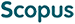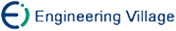丁立中 廖士中. KMA-α：一个支持向量机核矩阵的近似计算算法[J]. 计算机研究与发展, 2012, 49(4): 746-753.
 引用本文: 丁立中 廖士中. KMA-α：一个支持向量机核矩阵的近似计算算法[J]. 计算机研究与发展, 2012, 49(4): 746-753.Ding Lizhong and Liao Shizhong. KMA-α：A Kernel Matrix Approximation Algorithm for Support Vector Machines[J]. Journal of Computer Research and Development, 2012, 49(4): 746-753.
 Citation: Ding Lizhong and Liao Shizhong. KMA-α：A Kernel Matrix Approximation Algorithm for Support Vector Machines[J]. Journal of Computer Research and Development, 2012, 49(4): 746-753.## KMA-α：A Kernel Matrix Approximation Algorithm for Support Vector Machines

• 摘要: 核矩阵计算是求解支持向量机的关键，已有精确计算方法难以处理大规模的样本数据.为此，研究核矩阵的近似计算方法.首先，借助支持向量机的凸二次约束线性规划表示，给出支持向量机和多核支持向量机的二阶锥规划表示.然后，综合Monte Carlo方法和不完全Cholesky分解方法，提出一个新的核矩阵近似算法KMA-α，该算法首先对核矩阵进行Monte Carlo随机采样，采样后不直接进行奇异值分解，而是应用具有对称置换的不完全Cholesky分解来计算接近最优的低秩近似.以KMA-α输出的近似核矩阵作为支持向量机的输入，可提高支持向量机二阶锥规划求解的效率.进一步，分析了KMA-α的算法复杂性，证明了KMA-α的近似误差界定理.最后，通过标准数据集上的实验，验证了KMA-α的合理性和计算效率.理论分析与实验结果表明，KMA-α是一合理、有效的核矩阵近似算法.

Abstract: The computation of kernel matrices is essential for solving the support vector machines (SVM). Since the previous accurate approach is hard to apply in large-scale problems, there has been a lot deal of recent interests in the approximate approach, and a new approximation algorithm for the computation of kernel matrices is proposed in this paper. Firstly, we reformulate the quadratic optimization for SVM and multiple kernel SVM as a second-order cone programming (SOCP) through the convex quadratically constrained linear programming (QCLP). Then, we synthesize the Monte Carlo approximation and the incomplete Cholesky factorization, and present a new kernel matrix approximation algorithm KMA-α. KMA-α uses the Monte Carlo algorithm to randomly sample the kernel matrix. Rather than directly calculate the singular value decomposition of the sample matrix, KMA-α applies the incomplete Cholesky factorization with symmetric permutation to obtain the near-optimal low rank approximation of the sample matrix. The approximate matrix produced by KMA-α can be used in SOCP to improve the efficiency of SVM. Further, we analyze the computational complexity and prove the error bound theorem about the KMA-αalgorithm. Finally, by the comparative experiments on benchmark datasets, we verify the validity and the efficiency of KMA-α. Theoretical and experimental results show that KMA-α is a valid and efficient kernel matrix approximation algorithm./下载:  全尺寸图片 幻灯片
• 分享
• 用微信扫码二维码

分享至好友和朋友圈